##### The Japanese Bonsai specialist
Direct order Contact Help / Services Newsletter# Saxifraga fortunei 'beni tengu'

Saxifraga fortunei beni tengu daimonjiso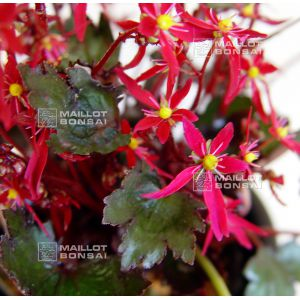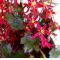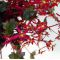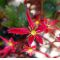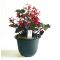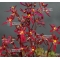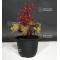ref. : 864711,50

Available quantity : 25Order

###### Description

Two-year-old plant delivered in a 0.8l plastic pot. Abundant flowers on 15 /25 cm stems. Height 10-25 cm, depending on the variety.

Saxifraga fortunei 'Beni Tengu' is a Japanese vivacious plant with delicate dark pink flowers and jagged dark green leaves with red undersides.

Flowers between mid-October and the end of November, depending on the variety and the arrival of cold weather.

The Japanese name for saxifraga fortunei is Daimonjiso. The flowers, with two long characteristic petals, resemble the 'Dai' written character, meaning 'great'. The full name is 'Great Letter Plant'.

Growing conditions: mid-shade to shady in slightly acidic, well drained soil. Don't let water stagnate at the foot or leave heaps of dead leaves that cause rot around the base. Can be grown in a pot (in a cool place safe from frost) and displayed as a kusamono to accompany bonsai. It can also be planted on low walls or in flowerbeds.

Maillot-Bonsai has been cultivating saxifrages for more than 10 years with great success. This species can resist cold temperatures of -15 to-18 degrees. Saxifrage lose their leaves in winter and grow again at the start of spring.

#saxifraga 4.9 #fortunei 4.8 #flowers 4.7 #with 4.4 #saxifrages 4 #leaves 3.6 #great 3.5 #tengu 3.5 #plant 3.5 #beni 3.4

Formule
(( ROUND((CHAR_LENGTH(b.article_nom)-CHAR_LENGTH(REPLACE(b.article_nom, 'saxifraga', '')))/LENGTH('saxifraga')) + ROUND((CHAR_LENGTH(b.article_description)-CHAR_LENGTH(REPLACE(b.article_description, 'saxifraga', '')))/LENGTH('saxifraga')) ) * 4.9) + (( ROUND((CHAR_LENGTH(b.article_nom)-CHAR_LENGTH(REPLACE(b.article_nom, 'fortunei', '')))/LENGTH('fortunei')) + ROUND((CHAR_LENGTH(b.article_description)-CHAR_LENGTH(REPLACE(b.article_description, 'fortunei', '')))/LENGTH('fortunei')) ) * 4.8) + (( ROUND((CHAR_LENGTH(b.article_nom)-CHAR_LENGTH(REPLACE(b.article_nom, 'flowers', '')))/LENGTH('flowers')) + ROUND((CHAR_LENGTH(b.article_description)-CHAR_LENGTH(REPLACE(b.article_description, 'flowers', '')))/LENGTH('flowers')) ) * 4.7) + (( ROUND((CHAR_LENGTH(b.article_nom)-CHAR_LENGTH(REPLACE(b.article_nom, 'with', '')))/LENGTH('with')) + ROUND((CHAR_LENGTH(b.article_description)-CHAR_LENGTH(REPLACE(b.article_description, 'with', '')))/LENGTH('with')) ) * 4.4) + (( ROUND((CHAR_LENGTH(b.article_nom)-CHAR_LENGTH(REPLACE(b.article_nom, 'leaves', '')))/LENGTH('leaves')) + ROUND((CHAR_LENGTH(b.article_description)-CHAR_LENGTH(REPLACE(b.article_description, 'leaves', '')))/LENGTH('leaves')) ) * 3.6) + (( ROUND((CHAR_LENGTH(b.article_nom)-CHAR_LENGTH(REPLACE(b.article_nom, 'plant', '')))/LENGTH('plant')) + ROUND((CHAR_LENGTH(b.article_description)-CHAR_LENGTH(REPLACE(b.article_description, 'plant', '')))/LENGTH('plant')) ) * 3.5) + (( ROUND((CHAR_LENGTH(b.article_nom)-CHAR_LENGTH(REPLACE(b.article_nom, 'tengu', '')))/LENGTH('tengu')) + ROUND((CHAR_LENGTH(b.article_description)-CHAR_LENGTH(REPLACE(b.article_description, 'tengu', '')))/LENGTH('tengu')) ) * 3.5) + (( ROUND((CHAR_LENGTH(b.article_nom)-CHAR_LENGTH(REPLACE(b.article_nom, 'great', '')))/LENGTH('great')) + ROUND((CHAR_LENGTH(b.article_description)-CHAR_LENGTH(REPLACE(b.article_description, 'great', '')))/LENGTH('great')) ) * 3.5) + (( ROUND((CHAR_LENGTH(b.article_nom)-CHAR_LENGTH(REPLACE(b.article_nom, 'beni', '')))/LENGTH('beni')) + ROUND((CHAR_LENGTH(b.article_description)-CHAR_LENGTH(REPLACE(b.article_description, 'beni', '')))/LENGTH('beni')) ) * 3.4) + (( ROUND((CHAR_LENGTH(b.article_nom)-CHAR_LENGTH(REPLACE(b.article_nom, 'depending', '')))/LENGTH('depending')) + ROUND((CHAR_LENGTH(b.article_description)-CHAR_LENGTH(REPLACE(b.article_description, 'depending', '')))/LENGTH('depending')) ) * 2.9)

## Secure payment## Delivery

Our logistic partners :